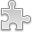# Courses / Module

Toggle Print

##PHYSICS FOR ENGINEERS 1

Module code: EP101EE
Credits: 5
Semester: 1
Department: EXPERIMENTAL PHYSICS
International:Coordinator: Dr Neil Trappe (EXPERIMENTAL PHYSICS)Overview

The aims of this module are:
* To introduce students to the physics of mechanics and heat, and to tutor them in the solution of problems in these two areas.
* To emphasize problem-solving: setting up diagrams of situations, setting up equilibrium equations, and solving these equations
Indicative Syllabus:

_Mechanics: (approx 18 lectures)
* Introduction and mathematical concepts; Vectors and vector components
* Linear Motion with Constant Acceleration; Projectile Motion
* Relative Velocity; Newton�s Laws of Motion and Engineering Applications
* Free body Diagrams, Equilibrium Equations
* Circular Motion, Centripetal Forces
* Work and Energy, Conservative and Non-conservative forces
* Impulse and Momentum, Law of Conservation of Momentum, Elastic and non-elastic collisions

_Heat: (approx 6 lectures)

* Temperature and heat, Thermal Expansion, Heat Capacity, Latent Heat
* The transfer of heat: Convection, Conduction, Radiation

Note: this module previously carried the EE104 code.Learning OutcomesTeaching & Learning methodsAssessmentAutumn Supplementals/ResitsAdditional ReadingTimetable